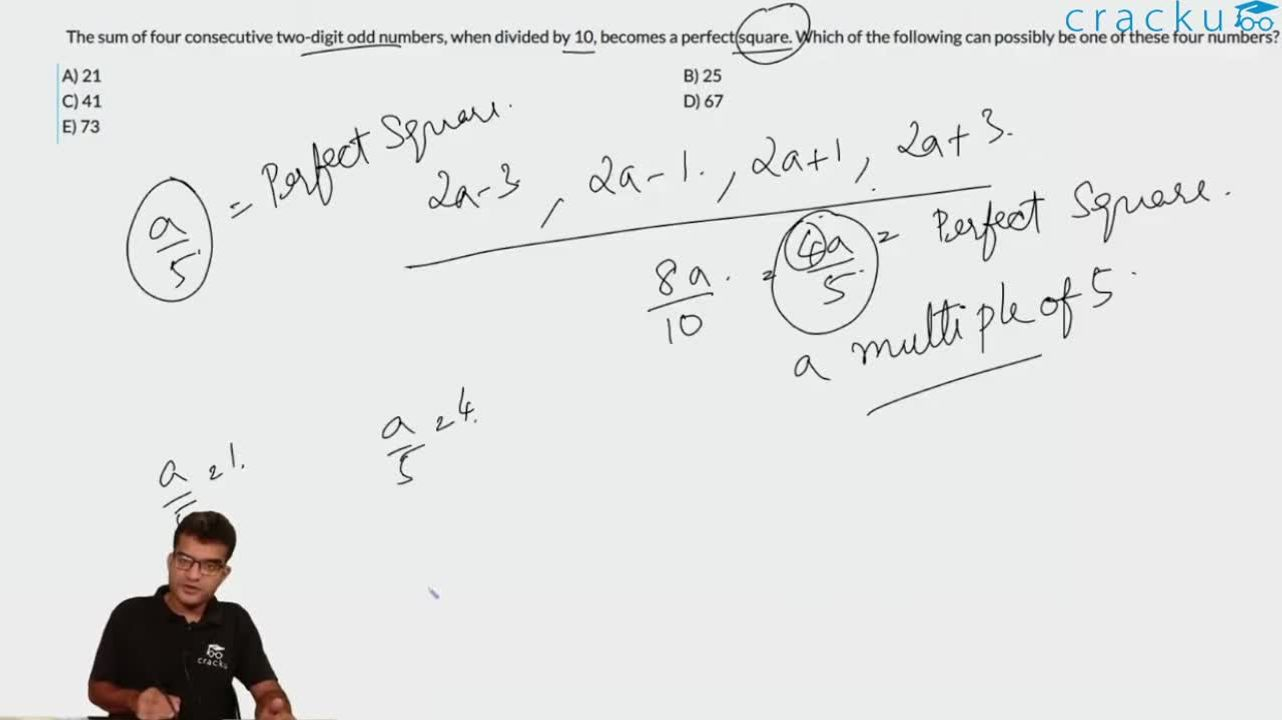Question 14

# The sum of four consecutive two-digit odd numbers, when divided by 10, becomes a perfect square. Which of the following can possibly be one of these four numbers?

Solution

Maximum sum of the four numbers <= 384=99+97+95+93
384/10 = 38.4
So, the perfect square is a number less than 38.4
The possibilities are 36, 25, 16 and 9
For the sum to be 360, the numbers can be 87, 89, 91 and 93
The sum of four consecutive odd numbers cannot be 250
For the sum to be 160, the numbers can be 37,39,41 and 43
The sum of 4 consecutive odd numbers cannot be 90
So, from the options, the answer is 41.

### View Video Solution